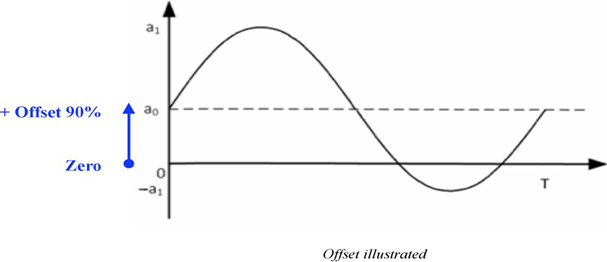# Offset

This is the imbalance between positive and negative peaks in reference to neutral or zero. Offsetting the signal from zero (neutral) means moving the waveform into the positive or negative region.You can visualize this by imagining horizontal X and vertical Y axis with sine wave crossing X. If both peaks are in equal distance (magnitude) from the X axis, then offset is zero.If we apply a positive DC offset to a sine wave, the wave will shift upwards - refer to the graphic below. Offset can have either positive or negative value.1 out of 1 found this helpful